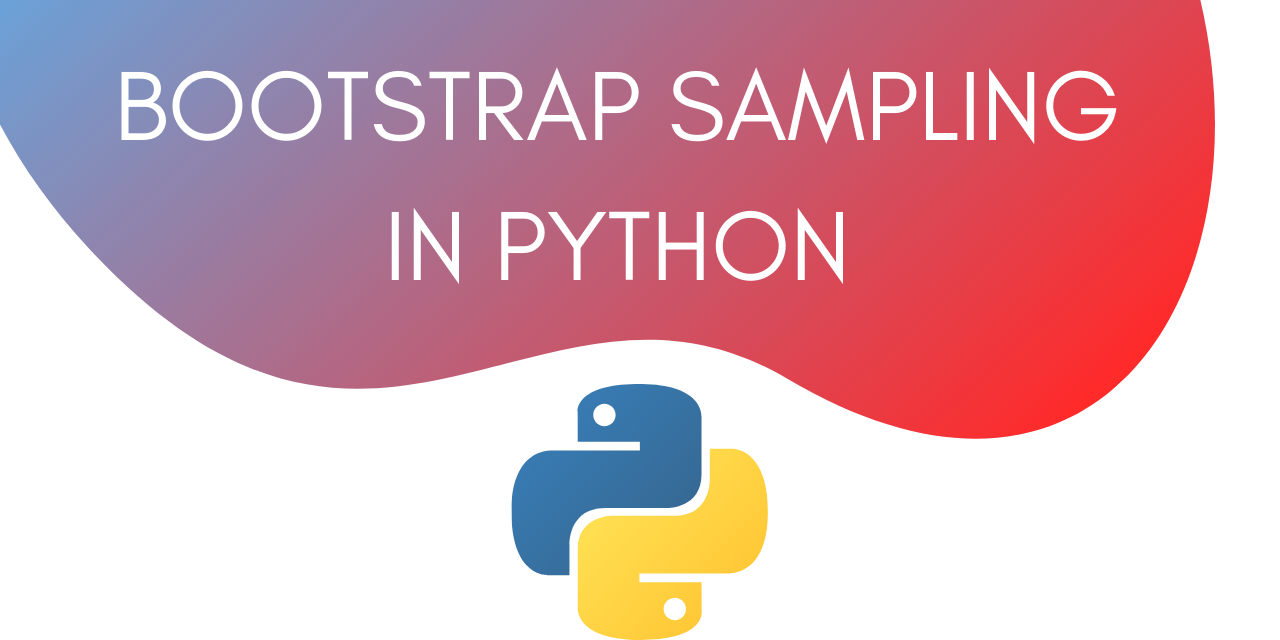# Bootstrap Sampling in Python

Published on August 3, 2022By Jayant VermaWhile we believe that this content benefits our community, we have not yet thoroughly reviewed it. If you have any suggestions for improvements, please let us know by clicking the “report an issue“ button at the bottom of the tutorial.

This is a tutorial on Bootstrap Sampling in Python. In this tutorial, we will learn what is bootstrapping and then see how to implement it.

Let’s get started.

## What is Bootstrap Sampling?

The definition for bootstrap sampling is as follows :

In statistics, Bootstrap Sampling is a method that involves drawing of sample data repeatedly with replacement from a data source to estimate a population parameter.

This basically means that bootstrap sampling is a technique using which you can estimate parameters like mean for an entire population without explicitly considering each and every data point in the population.

Instead of looking at the entire population, we look at multiple subsets all of the same size taken from the population.

For example, if your population size is 1000. Then to find the mean, instead of considering all the 1000 entries you can take 50 samples of size 4 each and calculate the mean for each sample. This way you will be taking an average of 200 entries (50X4) chosen randomly.

A similar strategy is used by market researchers to carry out research in a huge population.

## How to implement Bootstrap Sampling in Python?

Now let’s look at how to implement bootstrap sampling in python.

We will generate some random data with a predetermined mean. To do that we are going to use the NumPy module in Python.

Let’s start by importing the necessary modules.

### 1. Import the necessary modules.

The modules we need are :

• Numpy
• Random

To import these modules, use :

``````import numpy as np
import random
``````

In the next step, we need to generate some random data. Let’s do that using the Numpy module.

### 2. Generate Random Data

Let’s generate a normal distribution with a mean of 300 and with 1000 entries.

The code for that is given below:

``````x = np.random.normal(loc= 300.0, size=1000)
``````

We can calculate the mean of this data using :

``````print (np.mean(x))
``````

Output :

``````300.01293472373254
``````

Note that this is the actual mean of the population.

### 3. Use Bootstrap Sampling to estimate the mean

Let’s create 50 samples of size 4 each to estimate the mean.

The code for doing that is :

``````sample_mean = []

for i in range(50):
y = random.sample(x.tolist(), 4)
avg = np.mean(y)
sample_mean.append(avg)
``````

The list sample_mean will contain the mean for all the 50 samples. For estimating the mean of the population we need to calculate the mean for sample_mean.

You can do that using :

``````print(np.mean(sample_mean))
``````

Output :

``````300.07261467146867
``````

Now if we run the code in this section again then we will get a different output. This is because each time we run the code, we will generate new samples. However, each time the output will be close to the actual mean (300).

On running the code in this section again, we get the following output :

``````299.99137705245636
``````

Running it again, we get:

``````300.13411004148315
``````

## Complete code to Implement Bootstrap Sampling in Python

Here’s the complete code for this tutorial :

``````import numpy as np
import random

x = np.random.normal(loc= 300.0, size=1000)
print(np.mean(x))

sample_mean = []
for i in range(50):
y = random.sample(x.tolist(), 4)
avg = np.mean(y)
sample_mean.append(avg)

print(np.mean(sample_mean))
``````

## Conclusion

This tutorial was about Bootstrap Sampling in Python. We learned how to estimate the mean of a population by creating smaller samples. This is very useful in the world of Machine Learning to avoid overfitting. Hope you had fun learning with us!

Thanks for learning with the DigitalOcean Community. Check out our offerings for compute, storage, networking, and managed databases.Jayant Verma

author

#### Still looking for an answer?

Ask a questionSearch for more help

Click below to sign up and get \$200 of credit to try our products over 60 days!

### Popular Topics

##### Join the Tech Talk##### Hollie's Hub for Good

Working on improving health and education, reducing inequality, and spurring economic growth? We’d like to help.